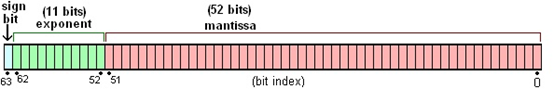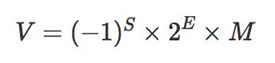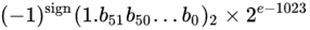# 置顶原理解析 | JavaScript 计算0.1 + 0.2真的很难，看完才知道！精选热门

• 浮点数十进制转二进制
• IEEE 754 标准
• 浮点数二进制运算
• 实践扩展

0.1的二进制：0.000110011......0011......(0011无限循环)

0.2的二进制：0.00110011......0011...... (0011无限循环)

IEEE 754 标准

IEEE二进制浮点数算术标准（IEEE 754）是20世纪80年代以来最广泛使用的浮点数运算标准，为许多CPU与浮点运算器所采用。S为符号位：表示浮点数的正负（0代表正数，1代表负数）；

E为指数位：存储指数，该数都会加上一个常数（偏移量），用来表示次方数；

M为尾数位：表示有效位（尾数），超出的部分自动进1舍0；0.1的二进制

0.000110011001100110011001100110011001100110011001100110011...... (0011无限循环)

0.1科学计数法表示

1.10011001100110011001100110011001100110011001100110011......(0011无限循环) * 2^-4

1.1001100110011001100110011001100110011001100110011010* 2^-4

0.1二进制存储格式

0,01111111011;1001100110011001100110011001100110011001100110011010

0.2的二进制

0.001100110011001100110011001100110011001100110011001100110011...... (0011无限循环)

0.2科学计数法表示

1.10011001100110011001100110011001100110011001100110011......(0011无限循环) * 2^-3

1.1001100110011001100110011001100110011001100110011010...... (0011无限循环) * 2^-3

0.2二进制存储格式

0,01111111100;1001100110011001100110011001100110011001100110011010

11001100110011001100110011001100110011001100110011010

——> 1100110011001100110011001100110011001100110011001101

0.1的科学计数法表示：

0.1100110011001100110011001100110011001100110011001101 *2^-3

0.1的二进制存储格式：

0,01111111100;1100110011001100110011001100110011001100110011001101

0.1的尾数 + 0.2的尾数 =

0.1100110011001100110011001100110011001100110011001101 1.1001100110011001100110011001100110011001100110011010 = 10.0110011001100110011001100110011001100110011001100111

0.1 + 0.2 = 10.0110011001100110011001100110011001100110011001100111* 2^-3

1.00110011001100110011001100110011001100110011001100111 * 2^-2

1.0011001100110011001100110011001100110011001100110100* 2^-2

0,01111111101;0011001100110011001100110011001100110011001100110100

1.0011001100110011001100110011001100110011001100110100* 2^-2

——>

0.010011001100110011001100110011001100110011001100110100

0.1 + 0.2 =

0.3000000000000000444089209850062616169452667236328125

```var_dump(number_format(0+ 1 * pow(2, -2) + 0 + 0 + 1 * pow(2, -5) + 1 * pow(2, -6) + 0 + 0 + 1 * pow(2,-9) + 1 * pow(2, -10) + 0 + 0 + 1 * pow(2, -13) + 1 * pow(2, -14) + 0 + 0 + 1 *pow(2, -17) + 1 * pow(2, -18) + 0 + 0 + 1 * pow(2, -21) + 1 * pow(2, -22) + 0 +0 + 1 * pow(2, -25) + 1 * pow(2, -26) + 0 + 0 + 1 * pow(2, -29) + 1 * pow(2,-30) + 0 + 0 + 1 * pow(2, -33) + 1 * pow(2, -34) + 0 + 0 + 1 * pow(2, -37) + 1* pow(2, -38) + 0 + 0 + 1 * pow(2, -41) + 1 * pow(2, -42) + 0 + 0 + 1 * pow(2,-45) + 1 * pow(2, -46) + 0 + 0 + 1 * pow(2, -49) + 1 * pow(2, -50) + 0 + 1 *pow(2, -52) + 0 + 0, 52));
```